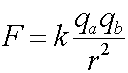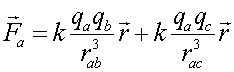The Formula Coulomb's law describes the force between two charged particles.Here, F is the force between the particles, qa and qb are the charges of particles a and b. The separation between the particles is r, and k is a constant, 8.99x109 (Nm2/C2). Note that the force falls off quadratically, similarly to the behavior of the gravitational force. The force is attractive, when F is negative, hence when the charges have opposite sign. Opposites attract - like charges repel. Of course, remember that force is a vector, which in this case points parallel to r. If a charge a is in the presence of several charges, the force that a feels is the sum of the forces from the remaining charges. For instance if there are three charges, a, b, and c, the net force felt by a is:where rab is the separation between a and b.